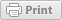Follow

# Data Insights Operators

Data Insights makes use of Relational operators to determine how particular filters are calculated. Queries can make use of multiple criteria to provide the best output.

Not all operators are available to all types of data, based off standard database querying standards.

The relational operators used in Data Insights are as follows:

 Operator: Used to: Data types where available: Equals Find all records where the value is X Text, Number, Date, Checkbox, Lookup List NOT equal to Find all records where the value is not X Text, Number, Date, Lookup List Greater than Find all records where the value is greater than X Number, Date Less than Find all records where the value is less than X Number, Date Greater than or equal to Find all records where the value is greater than or equal to X Number, Date Less than or equal to Find all records where the value is less than or equal to X Number, Date Starts with Find all records where the value starts with X Text Contains Find all records where the value contains X Text Is any of Find all records where the value is X or Y Lookup List Is NOT any of Find all records where the value is not X or Y Lookup List
Tags:
Was this article helpful?
0 out of 0 found this helpful
Have more questions? Submit a request

#### 0 Comments

Please sign in to leave a comment.